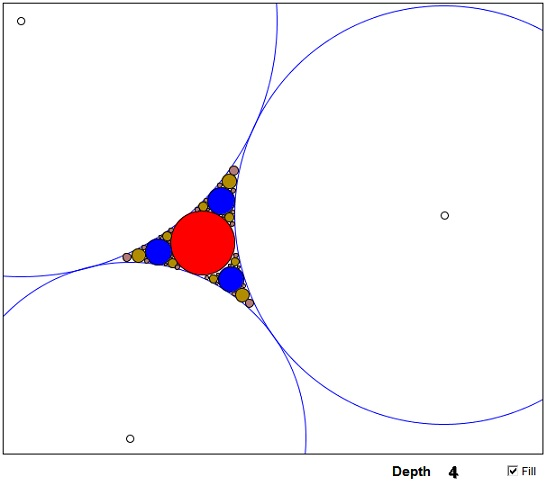Three touching circles form a curvelinear triangle. A unique circle exists that can be inscribed into that triangle. This is the inner Soddy circle. If the enterior of the Soddy circle is removed from the triangle, what remains is the union of three smaller curvelinear triangles. Each of those has a Soddy circle, whose removal leaves the union of three even smaller triangles, and so on. This process can continue indefinitely, not unlike the Trema removal procedure in the construction of Sierpinski's gasket. As in the linear case, the limit set, i.e., the set of points that remain from the orginal curvelinear triangle after an infinite number of steps is not empty. By analogy, it is called the Apollonian gasket, due to the obvious association with Apollonius' circles.

### If you are reading this, your browser is not set to run Java applets. Try IE11 or Safari and declare the site https://www.cut-the-knot.org as trusted in the Java setup.What if applet does not run?

The Apollonian gasket is an example (the Mandelbrot set is another one) of a fractal that is not self-similar. It is impossible therefore in its case to substitute the similarity dimension for the fractal dimension. The latter has to be evaluated directly from the Hausdorff-Besecovitch definition. Some numerical results are available. [Mandelbrot, p. 172] reports estimated bounds: the lower 1.300197 and the upper 1.314534.

### References

1. B. Mandelbrot, The Fractal Geometry of Nature, W.H.Freeman and Co., NY, 1977.•